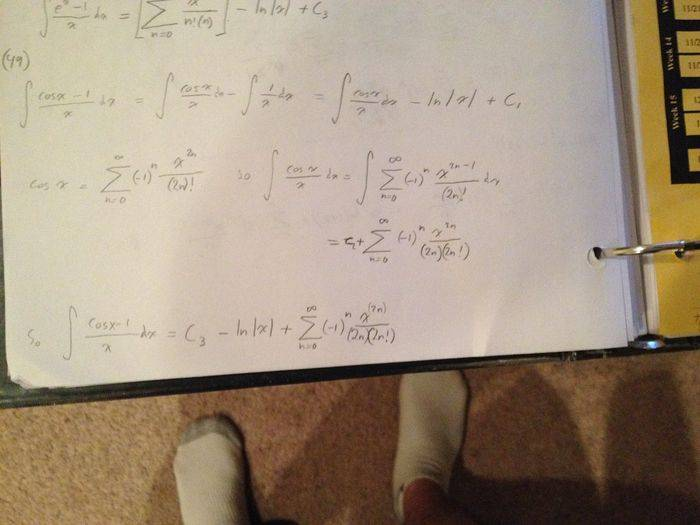# Taylor series integration of cosx -1 / x

Feodalherren

∫((cosX)-1)/x dx

Taylor Series

## The Attempt at a Solution

My approach was basically to to split the integral into two more manageable parts which gave me

∫(cosX/x)dx - ∫(1/x)dx

The solutions manual did it completely differently and instead changed the index of the sum to start from n=1 instead of n=0 which "ate up" the 1 on top. I guess my question is, did I do it wrong or is my solution correct, only not as pretty?

I got the same sum as they did, except mine starts at 0 and I have an extra natural log.

Homework Helper

∫((cosX)-1)/x dx

Taylor Series

## The Attempt at a Solution

My approach was basically to to split the integral into two more manageable parts which gave me

∫(cosX/x)dx - ∫(1/x)dx

The solutions manual did it completely differently and instead changed the index of the sum to start from n=1 instead of n=0 which "ate up" the 1 on top. I guess my question is, did I do it wrong or is my solution correct, only not as pretty?

I got the same sum as they did, except mine starts at 0 and I have an extra natural log.

You should really post what you actually did. The answer shouldn't have any natural logs at all. The Taylor series of cos(x) already has a 1 in it.

Feodalherren
I solved the two integrals: ∫(cosX/x)dx - ∫(1/x)dx.

The first one is exactly the same answer as the book got, except they shifted their index. I solved it by using the infinite sum definition of cosine. The integral of 1/x is ln x, that's where the log came from.

Homework Helper
I solved the two integrals: ∫(cosX/x)dx - ∫(1/x)dx.

The first one is exactly the same answer as the book got, except they shifted their index. I solved it by using the infinite sum definition of cosine. The integral of 1/x is ln x, that's where the log came from.

What did you do with the 1 in the series expansion of cos(x)? What do you think the series expansion of cos(x) is? These are the questions I'm trying to get at.

Homework Helper
Dearly Missed
I solved the two integrals: ∫(cosX/x)dx - ∫(1/x)dx.

The first one is exactly the same answer as the book got, except they shifted their index. I solved it by using the infinite sum definition of cosine. The integral of 1/x is ln x, that's where the log came from.

The integral of cos(x)/x is non-elementary, but it is expressible in terms of already-defined non-elementary functions. Is that what you did?

Feodalherren
Here's a picture of what I did. The light is kinda iffy but hopefully you'll see what's going on.Staff Emeritus
What you did is invalid. You have ##\frac{\cos x}{x} = \sum_{n=0}^{\infty} (-1)^n \frac{x^{2n-1}}{(2n)!}##. That's fine, but your integration of that isn't fine. Look at the n=0 term. You integrated that incorrectly.

Feodalherren
Hmm I'm not sure I understand what you mean. What term exactly are you referring to?

Staff Emeritus
The n=0 term of your sum, of course. You computed ##\int \frac{x^{2n-1}}{(2n!)}\,dx## as ##\frac{x^{2n}}{(2n)(2n!)}##. That is not valid when n=0.

•1 person
Mentor
Feodalherren, you're making this much harder than it needs to be. Do not split (cos(x) -1)/x into two terms. Write the Maclaurin series expansion of cos(x) - 1, preferably without the summation sign so that you can get the first few terms right.

Feodalherren
Ohh now I got it. Thanks.